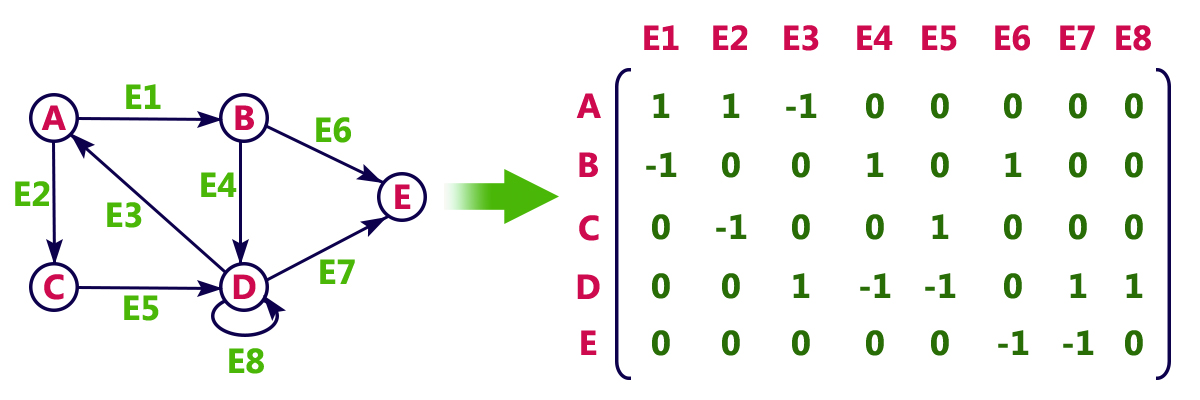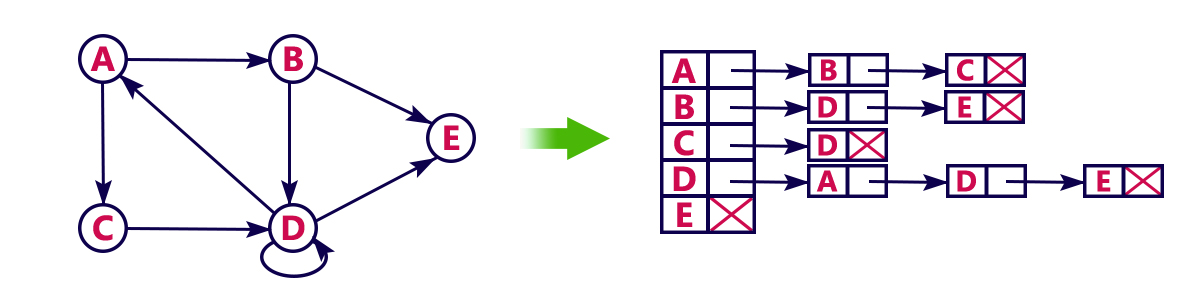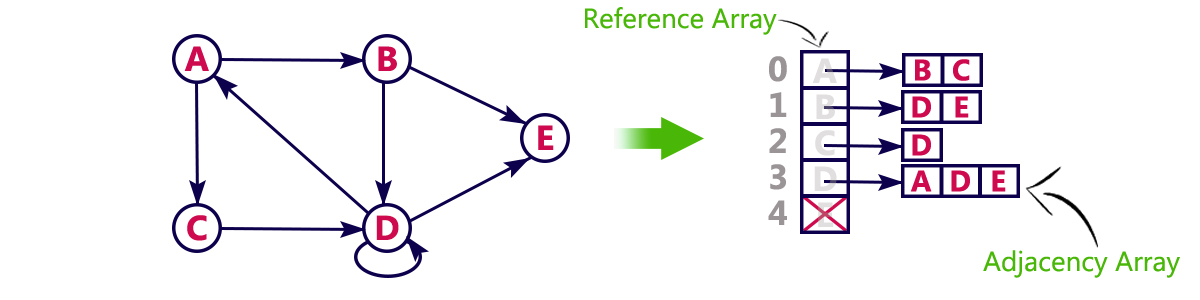The perfect place for easy learning...

×

# Graph Representations

Graph data structure is represented using following representations...

2. Incidence Matrix

In this representation, the graph is represented using a matrix of size total number of vertices by a total number of vertices. That means a graph with 4 vertices is represented using a matrix of size 4X4. In this matrix, both rows and columns represent vertices. This matrix is filled with either 1 or 0. Here, 1 represents that there is an edge from row vertex to column vertex and 0 represents that there is no edge from row vertex to column vertex.

For example, consider the following undirected graph representation...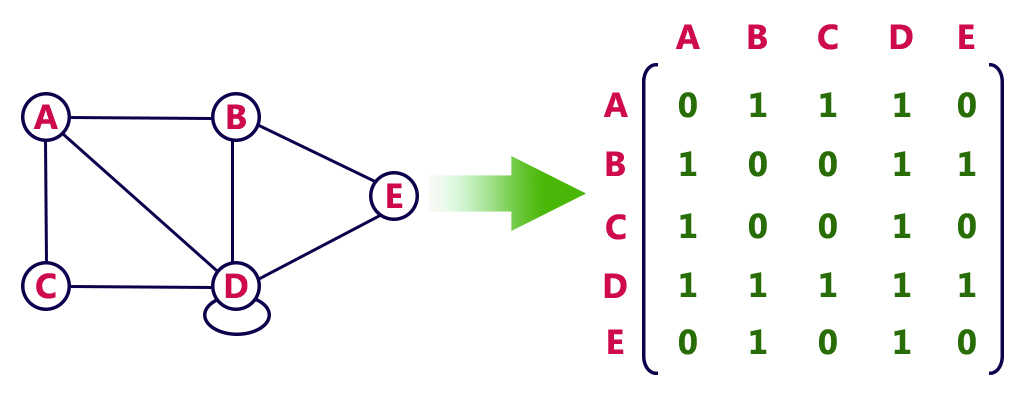Directed graph representation...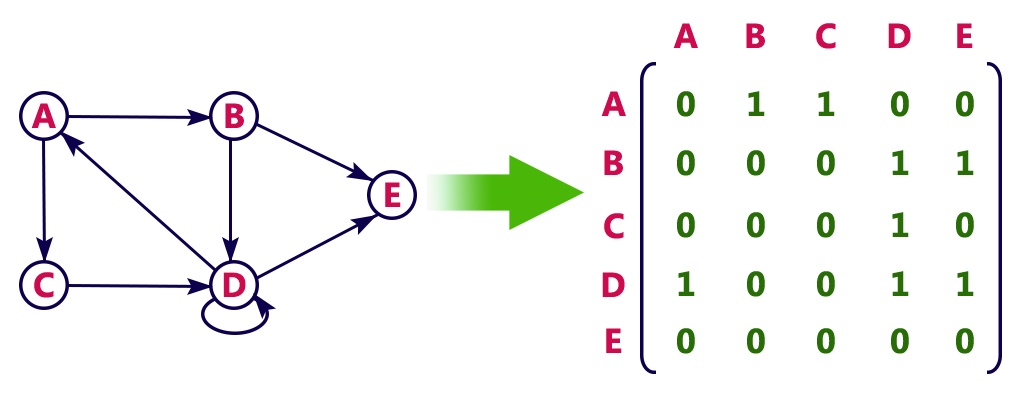# Incidence Matrix

In this representation, the graph is represented using a matrix of size total number of vertices by a total number of edges. That means graph with 4 vertices and 6 edges is represented using a matrix of size 4X6. In this matrix, rows represent vertices and columns represents edges. This matrix is filled with 0 or 1 or -1. Here, 0 represents that the row edge is not connected to column vertex, 1 represents that the row edge is connected as the outgoing edge to column vertex and -1 represents that the row edge is connected as the incoming edge to column vertex.

For example, consider the following directed graph representation...